Next: Multipole Expansion Up: Potential Theory Previous: Laplace's Equation in Spherical

# Poisson's Equation in Spherical Coordinates

Consider the general solution to Poisson's equation,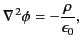(328)

in spherical coordinates. According to Section 2.3, the general three-dimensional Green's function for Poisson's equation is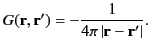(329)

When expressed in terms of spherical coordinates, this becomes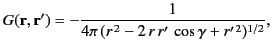(330)

where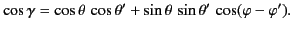(331)

is the angle subtended between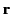and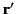. According to Equation (298), we can write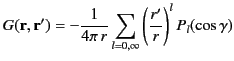(332)

for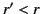, and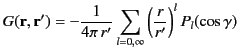(333)

for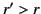. Thus, it follows from the spherical harmonic addition theorem, (322), that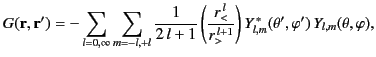(334)

where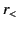represents the lesser of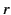and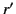, whereas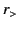represents the greater ofand.

According to Section 2.3, the general solution to Poisson's equation, (329), is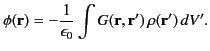(335)

Thus, Equation (335) yields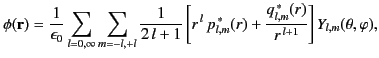(336)

where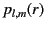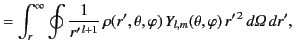(337)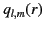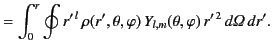(338)Next: Multipole Expansion Up: Potential Theory Previous: Laplace's Equation in Spherical
Richard Fitzpatrick 2014-06-27